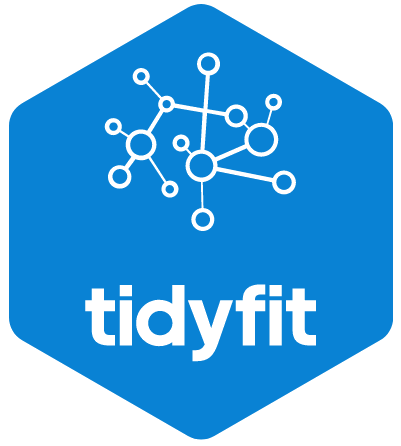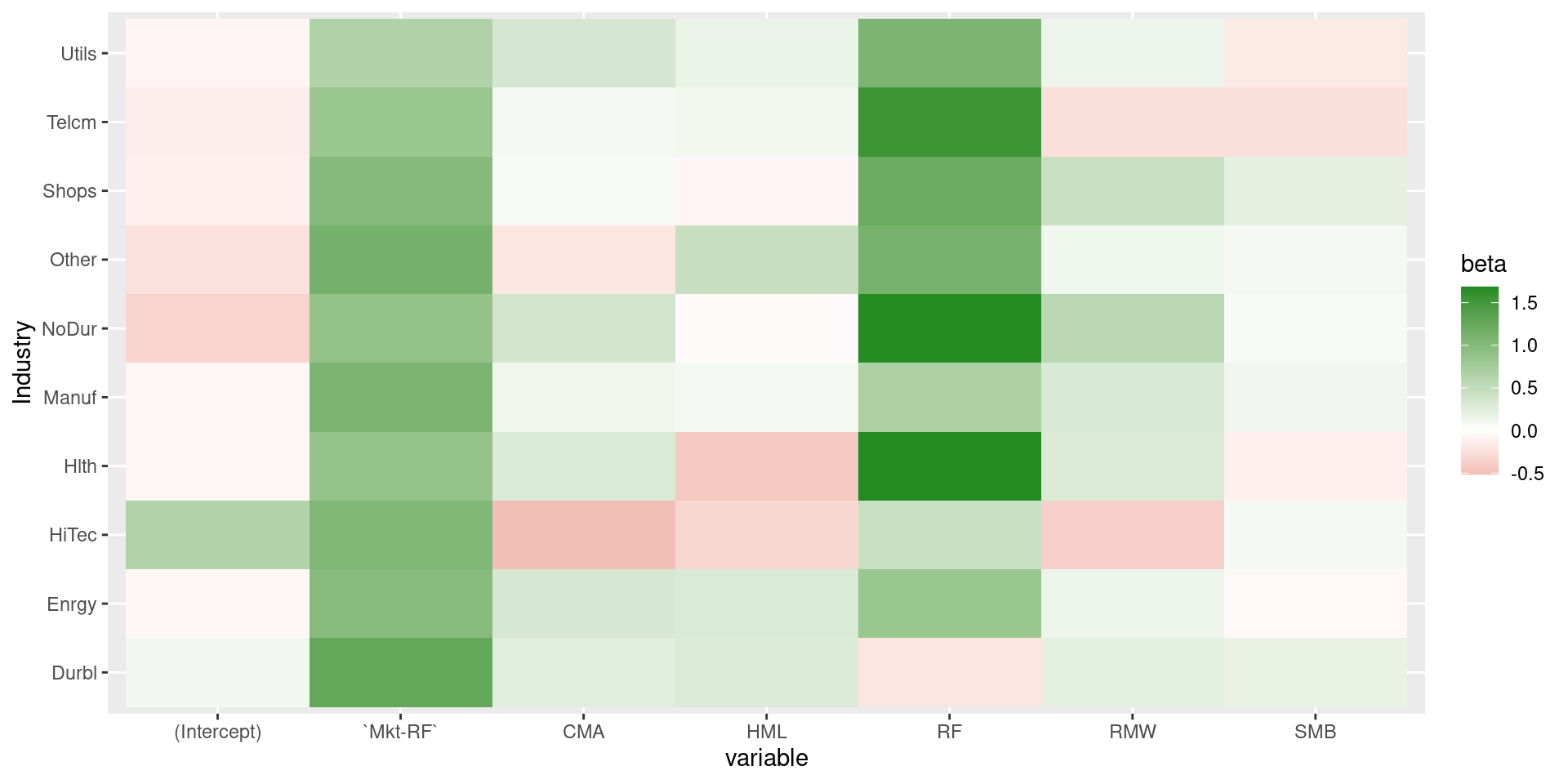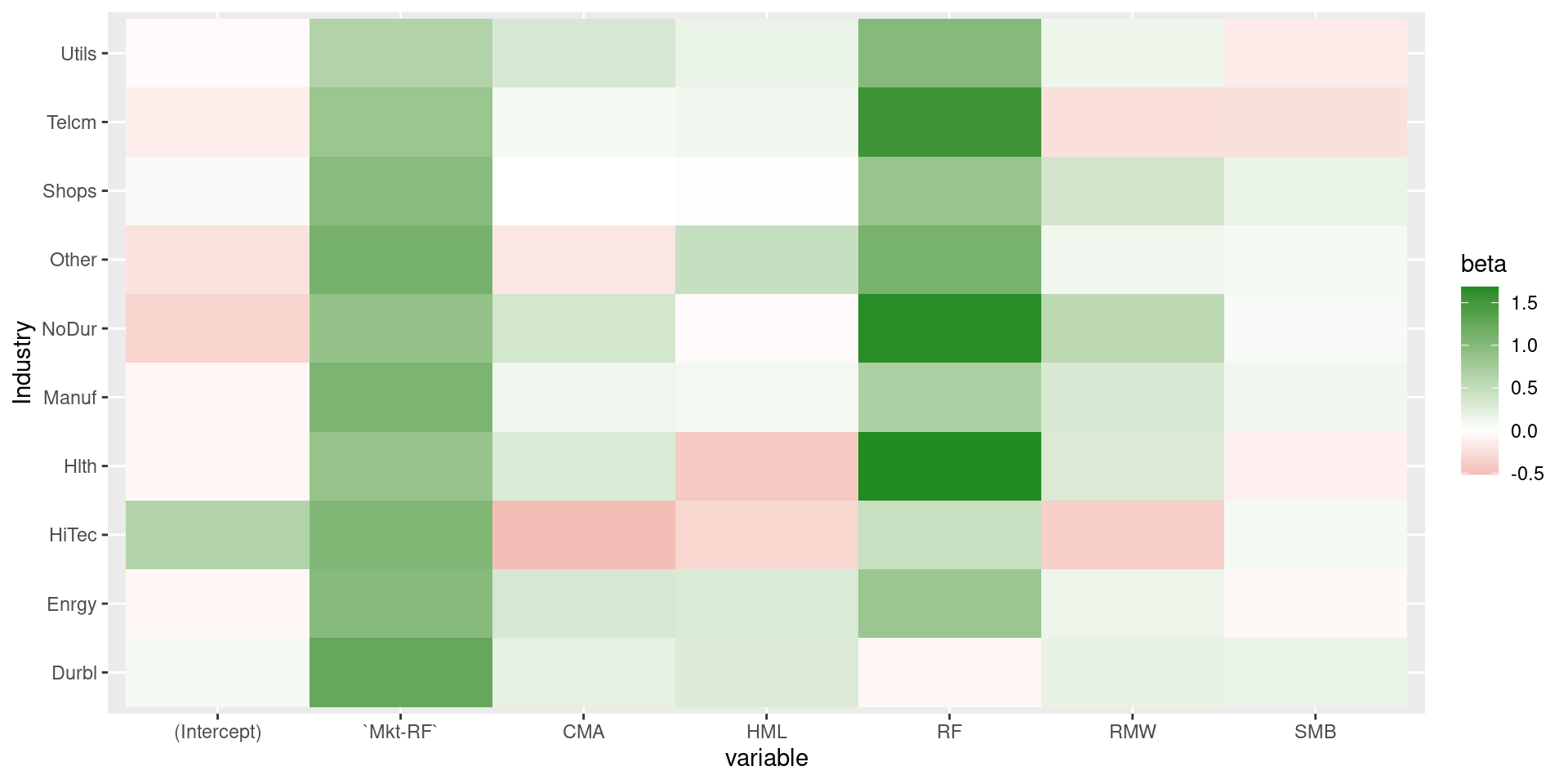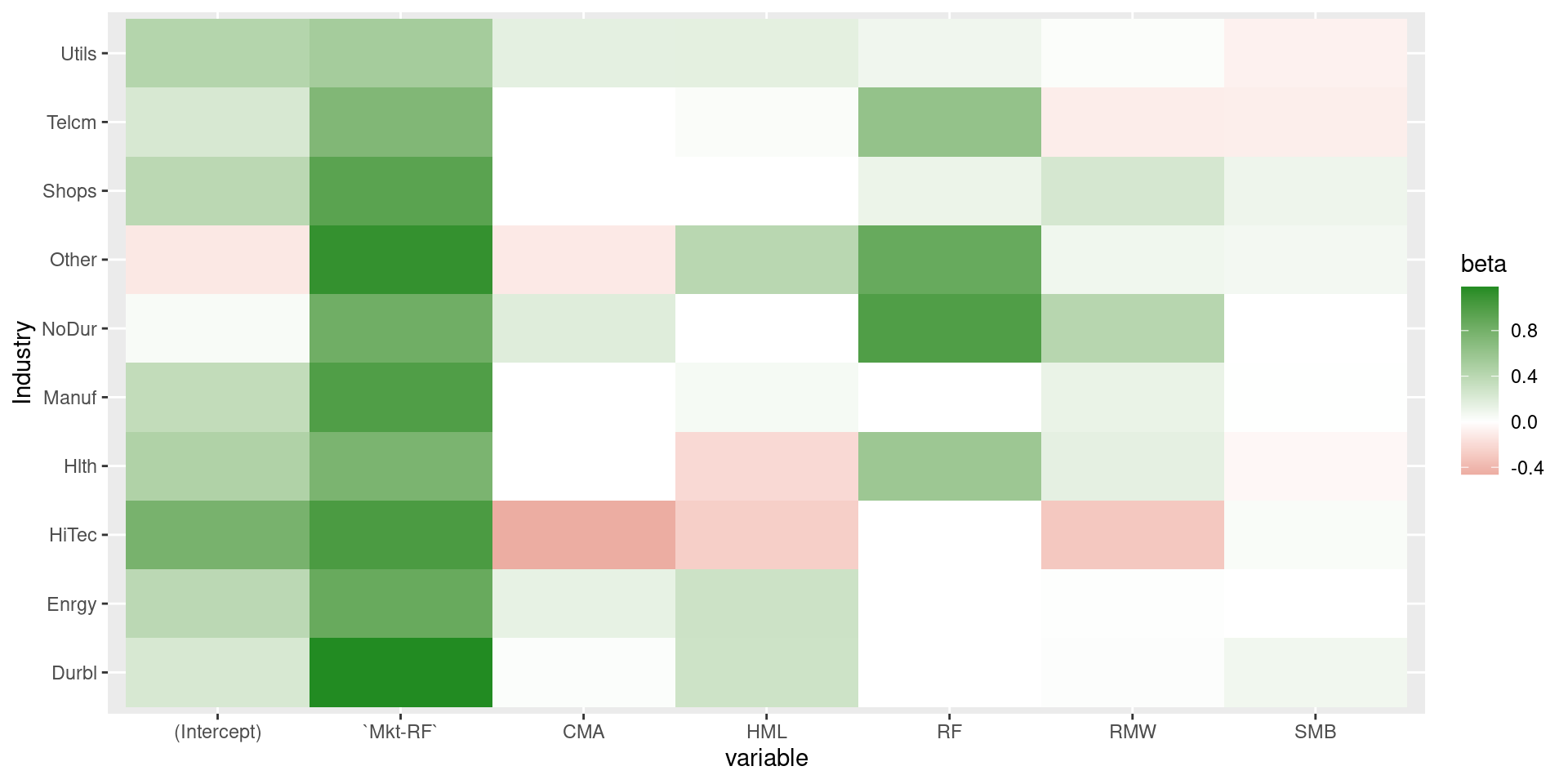# tidyfit: Extending the tidyverse with AutoMLtidyfit is an R-package that facilitates and automates linear regression and classification modeling in a tidy environment. The package includes several methods, such as Lasso, PLS and ElasticNet regressions, and can be augmented with custom methods. tidyfit builds on the tidymodels suite, but emphasizes automated modeling with a focus on the linear regression and classification coefficients, which are the primary output of tidyfit.

## Installation

You can install the development version of tidyfit from GitHub with:

# install.packages("devtools")
devtools::install_github("jpfitzinger/tidyfit")

## Example

### Fama-French factor and industry data

tidyfit includes a data set of financial factor returns freely available here. The data set includes monthly industry returns for 10 industries, as well as monthly factor returns for 5 factors:

library(tidyfit)
data <- tidyfit::Factor_Industry_Returns

### Fitting a linear regression

Models are fitted using tidyfit::tidyfit. Below a linear regression is fitted using the tidyfit::m.lm wrapper. The date columns is masked and the industry column is one-hot encoded:

fit <- data %>%
tidyfit(Return ~ ., lin_reg = m.lm, .mask = "Date")
fit
#> # A tibble: 16 × 4
#>    variable          beta model   model_info
#>    <chr>            <dbl> <chr>   <list>
#>  1 (Intercept)   -0.00408 lin_reg <tibble [1 × 4]>
#>  2 IndustryEnrgy -0.00409 lin_reg <tibble [1 × 4]>
#>  3 IndustryHiTec  0.0559  lin_reg <tibble [1 × 4]>
#>  4 IndustryHlth   0.0506  lin_reg <tibble [1 × 4]>
#>  5 IndustryManuf -0.0469  lin_reg <tibble [1 × 4]>
#>  6 IndustryNoDur  0.0171  lin_reg <tibble [1 × 4]>
#>  7 IndustryOther -0.0707  lin_reg <tibble [1 × 4]>
#>  8 IndustryShops  0.0405  lin_reg <tibble [1 × 4]>
#>  9 IndustryTelcm -0.184   lin_reg <tibble [1 × 4]>
#> 10 IndustryUtils -0.181   lin_reg <tibble [1 × 4]>
#> 11 CMA            0.117   lin_reg <tibble [1 × 4]>
#> 12 HML            0.0601  lin_reg <tibble [1 × 4]>
#> 13 Mkt-RF       0.977   lin_reg <tibble [1 × 4]>
#> 14 RF             1.01    lin_reg <tibble [1 × 4]>
#> 15 RMW            0.164   lin_reg <tibble [1 × 4]>
#> 16 SMB            0.0178  lin_reg <tibble [1 × 4]>

Detailed model and hyperparameter information is nested and can be expanded:

fit %>%
unnest(model_info)
#> # A tibble: 16 × 7
#>    variable          beta model      s.e. t value p value R.squared
#>    <chr>            <dbl> <chr>     <dbl>     <dbl>     <dbl>     <dbl>
#>  1 (Intercept)   -0.00408 lin_reg 0.133     -0.0306  9.76e- 1     0.625
#>  2 IndustryEnrgy -0.00409 lin_reg 0.172     -0.0237  9.81e- 1     0.625
#>  3 IndustryHiTec  0.0559  lin_reg 0.172      0.325   7.45e- 1     0.625
#>  4 IndustryHlth   0.0506  lin_reg 0.172      0.294   7.69e- 1     0.625
#>  5 IndustryManuf -0.0469  lin_reg 0.172     -0.272   7.85e- 1     0.625
#>  6 IndustryNoDur  0.0171  lin_reg 0.172      0.0994  9.21e- 1     0.625
#>  7 IndustryOther -0.0707  lin_reg 0.172     -0.411   6.81e- 1     0.625
#>  8 IndustryShops  0.0405  lin_reg 0.172      0.235   8.14e- 1     0.625
#>  9 IndustryTelcm -0.184   lin_reg 0.172     -1.07    2.85e- 1     0.625
#> 10 IndustryUtils -0.181   lin_reg 0.172     -1.05    2.93e- 1     0.625
#> 11 CMA            0.117   lin_reg 0.0281     4.18    2.94e- 5     0.625
#> 12 HML            0.0601  lin_reg 0.0182     3.31    9.30e- 4     0.625
#> 13 Mkt-RF       0.977   lin_reg 0.00985   99.3     0            0.625
#> 14 RF             1.01    lin_reg 0.145      6.99    2.91e-12     0.625
#> 15 RMW            0.164   lin_reg 0.0191     8.56    1.41e-17     0.625
#> 16 SMB            0.0178  lin_reg 0.0140     1.27    2.03e- 1     0.625

Now, instead of fitting a single regression, we need to fit a regression per industry. This is achieved simply by grouping:

fit <- data %>%
group_by(Industry) %>%
tidyfit(Return ~ ., lin_reg = m.lm, .mask = "Date")

fit %>%
ggplot(aes(variable, Industry)) +
geom_tile(aes(fill = beta)) +
scale_fill_gradient2(low = "firebrick", high = "forestgreen")### Fitting a Lasso regression

Fitting a Lasso regression requires hyperparameter tuning for the penalty lambda. This can be done by passing values to .cv and .cv_args. Cross validation is performed using rsample. See ?rsample::vfold_cv, ?rsample::loo_cv or ?rsample::rolling_origin for options that can be passed to .cv_args.

fit <- data %>%
group_by(Industry) %>%
tidyfit(Return ~ ., lasso_reg = m.lasso, .mask = "Date",
.cv = "vfold", .cv_args = list(v = 5))

fit %>%
ggplot(aes(variable, Industry)) +
geom_tile(aes(fill = beta)) +
scale_fill_gradient2(low = "firebrick", high = "forestgreen")The results do not appear to be different from a linear regression. To compare methods, simply pass multiple models:

fit <- data %>%
group_by(Industry) %>%
tidyfit(Return ~ ., lasso_reg = m.lasso, lin_reg = m.lm, .mask = "Date",
.cv = "vfold", .cv_args = list(v = 5))

Of course, a v-fold cross validation is not valid for ordered data. Instead simply set a rolling cross validation:

fit <- data %>%
group_by(Industry) %>%
tidyfit(Return ~ ., lasso_reg = m.lasso, .mask = "Date",
.cv = "ts", .cv_args = list(initial = 60, assess = 24, skip = 24, cumulative = FALSE))

fit %>%
ggplot(aes(variable, Industry)) +
geom_tile(aes(fill = beta)) +
scale_fill_gradient2(low = "firebrick", high = "forestgreen")### Predicting with an ElasticNet classifier

Let’s predict out-of-sample return probabilities:

data_train <- data %>%
mutate(Return = ifelse(Return > 0, 1, 0)) %>%
filter(Date <= 202112)

data_test <- data %>%
mutate(Return = ifelse(Return > 0, 1, 0)) %>%
filter(Date > 202112)

Classification is possible with tidyfit by passing family = "binomial" to .control:

fit <- data_train %>%
mutate(Return = ifelse(Return > 0, 1, 0)) %>%
group_by(Industry) %>%
tidyfit(Return ~ ., enet_clf = m.enet, .mask = "Date",
.cv = "ts", .cv_args = list(initial = 60, assess = 24, skip = 24, cumulative = FALSE),
.control = list(family = "binomial"))

Predictions can be made for all models using tidypredict:

pred <- fit %>%
tidypredict(data_test) %>%
mutate(Predicted = ifelse(pred > 0.5, 1, 0)) %>%
rename(Truth = Return)

# Print a confusion matrix
table(pred$Truth, pred$Predicted)
#>
#>      0  1
#>   0  5 13
#>   1  0 12

tidyfit makes a few things a little easier:

• Methods returned statistically comparable outputs. For instance, all covariates are standardized and the coefficients are back-transformed to the original scale. This is not done by all underlying packages (e.g. pls, which is used for the PCR and PLSR methods).
• Hyperparameter grids are set to reasonable starting values. Custom grids can be passed to .control (e.g. .control = list(lambda = c(seq(0, 1, by = 0.1)))).
• Hyperparameter can be tuned across all groups or separately within each group by setting the .tune_each_group flag.
• Results for the individual slices can be returned using the .return_slices flag. This is particularly useful for rolling window estimation, which can be done by returning the results of a rolling cross validation.